Biofloc fish project monitoring.

IntermediateWork in progressOver 2 days1,017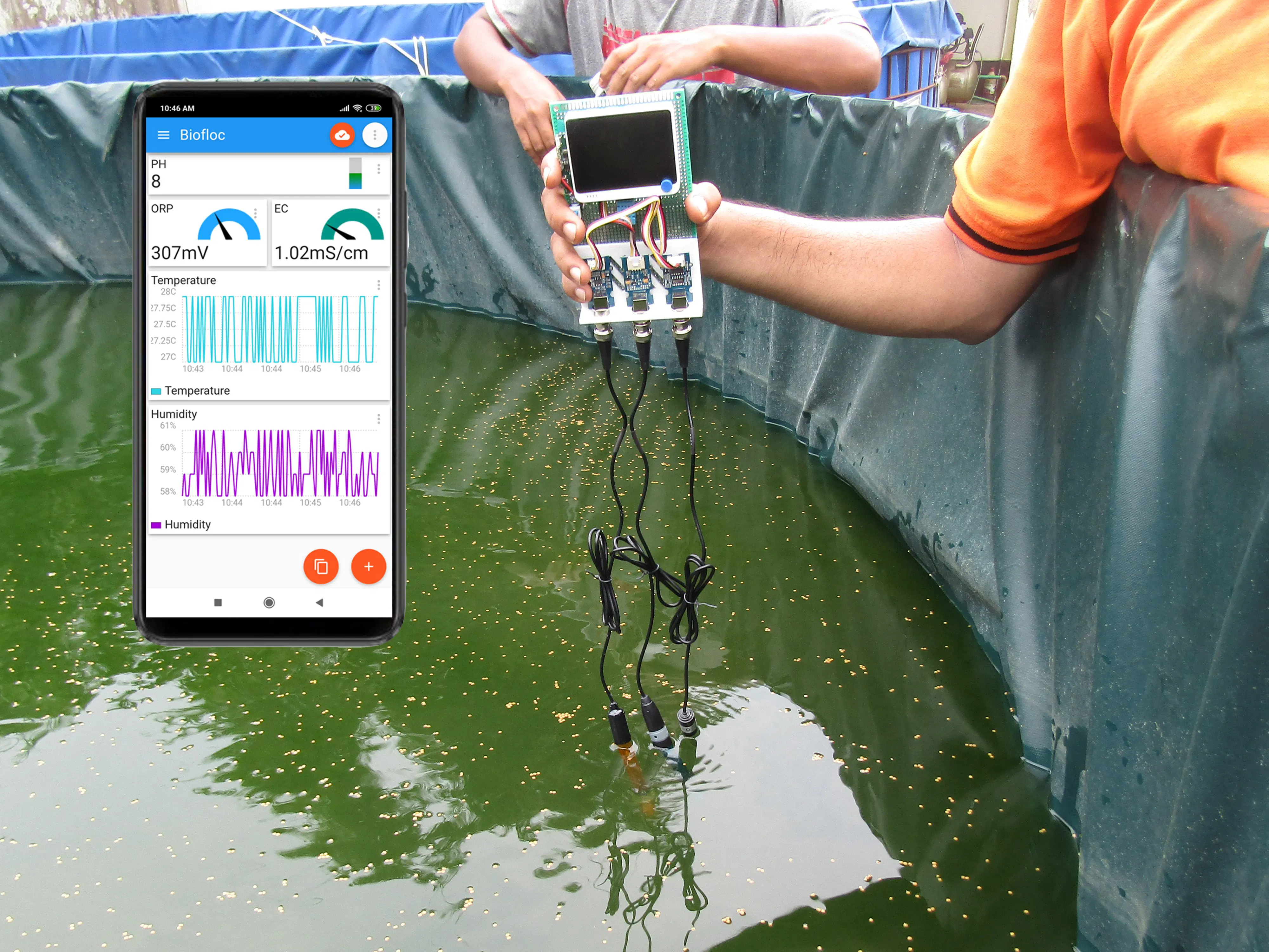## Things used in this project

### Hardware components

 Seeed Wio Terminal
×1
 Seeed Grove ORP Sensor
×1
 Seeed Grove PH Sensor
×1
 Seeed Grove EC Sensor
×1
 Seeed Grove DHT11 Sensor
×1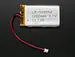Li-Ion Battery 1000mAh
×1
 SparkFun LiPower - Boost Converter
×1

### Software apps and online servicesArduino IDEMQTT

### Hand tools and fabrication machinesSoldering iron (generic)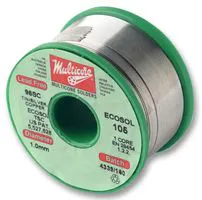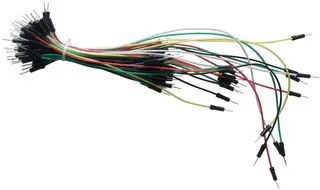65 Pc. Jumper Wire with Tips Assortment, 22 AWG3D Printer (generic)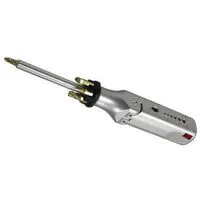Multitool, Screwdriver

## Schematics

### Schematic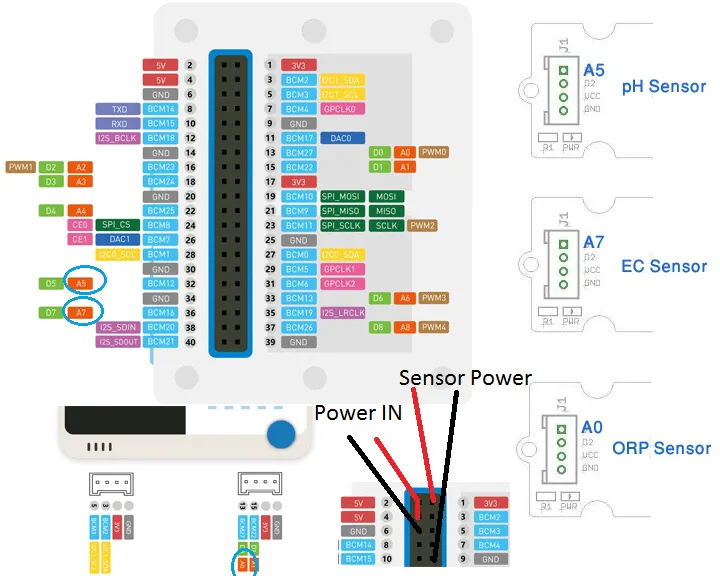## Code

### Arduino Sketch

Arduino
```#include "DHT.h"
#include "DFRobot_EC.h"
#include <EEPROM.h>
#include <AtWiFi.h>
#include <PubSubClient.h>
#define VOLTAGE 5.00    //system voltage
#define OFFSET 0        //zero drift voltage
#define PhSensorPin A5            //pH meter Analog output to Arduino Analog Input 5
#define EcSensorPin A7            //pH meter Analog output to Arduino Analog Input 7
#define OrpSensorPin A0            //pH meter Analog output to Arduino Analog Input 0
#define DHTPIN 8     // Digital pin connected to the DHT sensor
#define DHTTYPE DHT11   // DHT 11

#define Offset 41.02740741      //deviation compensate
#define samplingInterval 50

#define ArrayLenth  40    //times of collection

int pHArray[ArrayLenth];   //Store the average value of the sensor feedback
int pHArrayIndex = 0;

int orpArray[ArrayLenth];
int orpArrayIndex=0;

float voltage, ecValue, pHValue, orpValue, temperature, humidity;
DFRobot_EC ec;
DHT dht(DHTPIN, DHTTYPE);

#include"TFT_eSPI.h"
TFT_eSPI tft;

#define TFT_BLACK       0x0000      /*   0,   0,   0 */
#define TFT_NAVY        0x000F      /*   0,   0, 128 */
#define TFT_DARKGREEN   0x03E0      /*   0, 128,   0 */
#define TFT_DARKCYAN    0x03EF      /*   0, 128, 128 */
#define TFT_MAROON      0x7800      /* 128,   0,   0 */
#define TFT_PURPLE      0x780F      /* 128,   0, 128 */
#define TFT_OLIVE       0x7BE0      /* 128, 128,   0 */
#define TFT_LIGHTGREY   0xC618      /* 192, 192, 192 */
#define TFT_DARKGREY    0x7BEF      /* 128, 128, 128 */
#define TFT_BLUE        0x001F      /*   0,   0, 255 */
#define TFT_GREEN       0x07E0      /*   0, 255,   0 */
#define TFT_CYAN        0x07FF      /*   0, 255, 255 */
#define TFT_RED         0xF800      /* 255,   0,   0 */
#define TFT_MAGENTA     0xF81F      /* 255,   0, 255 */
#define TFT_YELLOW      0xFFE0      /* 255, 255,   0 */
#define TFT_WHITE       0xFFFF      /* 255, 255, 255 */
#define TFT_ORANGE      0xFDA0      /* 255, 180,   0 */
#define TFT_GREENYELLOW 0xB7E0      /* 180, 255,   0 */
#define TFT_GREY        0x5AEB

#define RED2RED 0
#define GREEN2GREEN 1
#define BLUE2BLUE 2
#define BLUE2RED 3
#define GREEN2RED 4
#define RED2GREEN 5

uint32_t runTime = -99999;       // time for next update
float reading = 0; // Value to be displayed
int d = 0; // Variable used for the sinewave test waveform

float     ltx     = 0;          // Saved x coord of bottom of needle
float     ltx1     = 0;          // Saved x coord of bottom of needle
uint16_t  osx     = 120, osy = 120;   // Saved x & y coords
uint16_t  osx1     = 120, osy1 = 240;   // Saved x & y coords
uint32_t  updateTime  = 0;            // time for next update

int old_analog  = -999;           // Value last displayed
int old_digital = -999;           // Value last displayed

int value   = {0, 0, 0, 0, 0, 0};
int old_value = { -1, -1, -1, -1, -1, -1};
//int d = 0;

int screen_select_flag = 1;

// Update these with values suitable for your network.
const char* ssid = "***"; // WiFi Name
const char* mqtt_server = "broker.mqtt-dashboard.com";  // MQTT Broker URL

WiFiClient wioClient;
PubSubClient client(wioClient);

void setup_wifi() {

delay(10);
tft.setTextSize(2);
tft.setCursor((320 - tft.textWidth("Connecting to Wi-Fi..")) / 2, 120);
tft.print("Connecting to Wi-Fi..");

Serial.println();
Serial.print("Connecting to ");
Serial.println(ssid);

while (WiFi.status() != WL_CONNECTED) {
delay(500);
Serial.print(".");
}

Serial.println("");
Serial.println("WiFi connected");

tft.fillScreen(TFT_BLACK);
tft.setCursor((320 - tft.textWidth("Connected!")) / 2, 120);
tft.print("Connected!");

Serial.println(WiFi.localIP()); // Display Local IP Address
}

void callback(char* topic, byte* payload, unsigned int length) {
//tft.fillScreen(TFT_BLACK);
Serial.print("Message arrived [");
Serial.print(topic);
Serial.print("] ");
char buff_p[length];
for (int i = 0; i < length; i++) {
}
Serial.println();

}

void reconnect() {
// Loop until we're reconnected
while (!client.connected()) {
Serial.print("Attempting MQTT connection...");
// Create a random client ID
String clientId = "WioTerminal-";
clientId += String(random(0xffff), HEX);
// Attempt to connect
if (client.connect(clientId.c_str())) {
Serial.println("connected");
// Once connected, publish an announcement...
client.publish("WTout", "hello world");
// ... and resubscribe
client.subscribe("WTin");
} else {
Serial.print("failed, rc=");
Serial.print(client.state());
Serial.println(" try again in 5 seconds");
// Wait 5 seconds before retrying
delay(5000);
}
}
}

void setup() {
dht.begin();
ec.begin();
tft.begin();
tft.setRotation(3);
tft.fillScreen(TFT_BLACK); //Black background
pinMode(WIO_KEY_A, INPUT_PULLUP);
pinMode(WIO_KEY_B, INPUT_PULLUP);

setup_wifi();
client.setServer(mqtt_server, 1883); // Connect the MQTT Server
client.setCallback(callback);

updateTime = millis(); // Next update time
}

void loop() {

static unsigned long samplingTime = millis();
static unsigned long printTime = millis();
static float  voltage;

if (millis() - samplingTime > samplingInterval)
{
calculate_orp_value();
calculate_ph_value();
calculate_ec_value();
samplingTime = millis();
}

display_information();

if (!client.connected()) {
reconnect();
}
client.loop();

long now = millis();
if (now - lastMsg > 50000) {
lastMsg = now;

Serial.print("Publish message: ");
client.publish("biofloc/temp", String(temperature).c_str());
client.publish("biofloc/humid", String(humidity).c_str());
client.publish("biofloc/orp", String(orpValue).c_str());
client.publish("biofloc/ec", String(ecValue).c_str());
client.publish("biofloc/ph", String(pHValue).c_str());
}
}

void top_analog_meter(){

// Meter outline
tft.fillRect(0, 0, 220, 120, TFT_CYAN);
tft.fillRect(5, 3, 211, 113, TFT_WHITE);

tft.setTextColor(TFT_BLACK);  // Text colour

// Draw ticks every 5 degrees from -50 to +50 degrees (100 deg. FSD swing)
for (int i = -50; i < 51; i += 5) {
// Long scale tick length
int tl = 15;

// Coodinates of tick to draw
float sx = cos((i - 98) * 0.0174532925); //98 is the left starting of the scale
float sy = sin((i - 98) * 0.0174532925);
uint16_t x0 = sx * (100 + tl) + 120; // drawing color
uint16_t y0 = sy * (100 + tl) + 140; //
uint16_t x1 = sx * 100 + 120; //
uint16_t y1 = sy * 100 + 140; //

// Coordinates of next tick for zone fill
float sx2 = cos((i + 5 - 98) * 0.0174532925);
float sy2 = sin((i + 5 - 98) * 0.0174532925);
int x2 = sx2 * (100 + tl) + 120;
int y2 = sy2 * (100 + tl) + 140;
int x3 = sx2 * 100 + 120;
int y3 = sy2 * 100 + 140;

// Yellow zone limits
if (i >= -50 && i < 0) {
tft.fillTriangle(x0, y0, x1, y1, x2, y2, TFT_YELLOW);
tft.fillTriangle(x1, y1, x2, y2, x3, y3, TFT_YELLOW);
}

// Green zone limits
if (i >= 0 && i < 25) {
tft.fillTriangle(x0, y0, x1, y1, x2, y2, TFT_GREEN);
tft.fillTriangle(x1, y1, x2, y2, x3, y3, TFT_GREEN);
}

// Orange zone limits
if (i >= 25 && i < 50) {
tft.fillTriangle(x0, y0, x1, y1, x2, y2, TFT_ORANGE);
tft.fillTriangle(x1, y1, x2, y2, x3, y3, TFT_ORANGE);
}

// Short scale tick length
if (i % 25 != 0) tl = 8;

// Recalculate coords incase tick lenght changed
x0 = sx * (100 + tl) + 120; //value of 100 is y pos of scale
y0 = sy * (100 + tl) + 140;
x1 = sx * 100 + 120;
y1 = sy * 100 + 140;

// Draw tick
tft.drawLine(x0, y0, x1, y1, TFT_BLACK);

// Check if labels should be drawn, with position tweaks
if (i % 25 == 0) {
// Calculate label positions
x0 = sx * (100 + tl + 10) + 120;
y0 = sy * (100 + tl + 10) + 140;
switch (i / 25) {
case -2: tft.drawCentreString( "0", x0, y0 - 12, 2); break;
case -1: tft.drawCentreString("5", x0, y0 -  9, 2); break;
case  0: tft.drawCentreString("10", x0, y0 -  6, 2); break;
case  1: tft.drawCentreString("15", x0, y0 -  9, 2); break;
case  2: tft.drawCentreString("20",x0, y0 - 12, 2); break;
}
}

// Now draw the arc of the scale
sx = cos((i + 5 - 98) * 0.0174532925);
sy = sin((i + 5 - 98) * 0.0174532925);
x0 = sx * 100 + 120;//120
y0 = sy * 100 + 140;//140
// Draw scale arc, don't draw the last part the arc below the scale
//if (i < 50) tft.drawLine(x0, y0, x1, y1, TFT_BLACK);
if (i < 50) tft.drawLine(x0, y0, x1, y1, TFT_BLACK);
}

tft.drawString("pH", 5 + 220 - 40, 100 - 20, 2);               // Units at bottom right
tft.drawCentreString("pH", 110, 70, 4);                    // Comment out to avoid font 4
tft.drawRect(5, 3, 211, 113, TFT_BLACK);                    // Draw bezel line
}

void bottom_analog_meter(){

// Meter outline
tft.fillRect(0, 120, 220, 240, TFT_CYAN); //x, y, x, y
tft.fillRect(5, 123, 211, 114, TFT_WHITE);

tft.setTextColor(TFT_BLACK);  // Text colour

// Draw ticks every 5 degrees from -50 to +50 degrees (100 deg. FSD swing)
for (int i = -50; i < 51; i += 5) {
// Long scale tick length
int tl = 15;

// Coodinates of tick to draw
float sx = cos((i - 98) * 0.0174532925); //98 is the left starting of the scale
float sy = sin((i - 98) * 0.0174532925);
uint16_t x0 = sx * (100 + tl) + 120; // drawing color
uint16_t y0 = sy * (100 + tl) + 260; //
uint16_t x1 = sx * 100 + 120; //
uint16_t y1 = sy * 100 + 260; //

// Coordinates of next tick for zone fill
float sx2 = cos((i + 5 - 98) * 0.0174532925);
float sy2 = sin((i + 5 - 98) * 0.0174532925);
int x2 = sx2 * (100 + tl) + 120;
int y2 = sy2 * (100 + tl) + 260;
int x3 = sx2 * 100 + 120;
int y3 = sy2 * 100 + 260;

// Yellow zone limits
if (i >= -50 && i < 0) {
tft.fillTriangle(x0, y0, x1, y1, x2, y2, TFT_YELLOW);
tft.fillTriangle(x1, y1, x2, y2, x3, y3, TFT_YELLOW);
}

// Green zone limits
if (i >= 0 && i < 25) {
tft.fillTriangle(x0, y0, x1, y1, x2, y2, TFT_GREEN);
tft.fillTriangle(x1, y1, x2, y2, x3, y3, TFT_GREEN);
}

// Orange zone limits
if (i >= 25 && i < 50) {
tft.fillTriangle(x0, y0, x1, y1, x2, y2, TFT_ORANGE);
tft.fillTriangle(x1, y1, x2, y2, x3, y3, TFT_ORANGE);
}

// Short scale tick length
if (i % 25 != 0) tl = 8;

// Recalculate coords incase tick lenght changed
x0 = sx * (100 + tl) + 120; //value of 100 is y pos of scale
y0 = sy * (100 + tl) + 260;
x1 = sx * 100 + 120;
y1 = sy * 100 + 260;

// Draw tick
tft.drawLine(x0, y0, x1, y1, TFT_BLACK);

// Check if labels should be drawn, with position tweaks
if (i % 25 == 0) {
// Calculate label positions
x0 = sx * (100 + tl + 10) + 120;
y0 = sy * (100 + tl + 10) + 260;
switch (i / 25) {
case -2: tft.drawCentreString( "0", x0, y0 - 12, 2); break;
case -1: tft.drawCentreString("50", x0, y0 -  9, 2); break;
case  0: tft.drawCentreString("100", x0, y0 -  6, 2); break;
case  1: tft.drawCentreString("150", x0, y0 -  9, 2); break;
case  2: tft.drawCentreString("200",x0, y0 - 12, 2); break;
}
}

// Now draw the arc of the scale
sx = cos((i + 5 - 98) * 0.0174532925);
sy = sin((i + 5 - 98) * 0.0174532925);
x0 = sx * 100 + 120;//120
y0 = sy * 100 + 260;//140
// Draw scale arc, don't draw the last part the arc below the scale
//if (i < 50) tft.drawLine(x0, y0, x1, y1, TFT_BLACK);
if (i < 50) tft.drawLine(x0, y0, x1, y1, TFT_BLACK);
}

tft.drawString("ORP", 5 + 220 - 40, 220 - 20, 2);               // Units at bottom right
tft.drawCentreString("ORP", 110, 200, 4);                    // Comment out to avoid font 4
tft.drawRect(5, 123, 211, 113, TFT_BLACK);                    // Draw bezel line
}

void plot_top_needle(int value, byte ms_delay){
//uint16_t  osx     = 120, osy = 120;
tft.setTextColor(TFT_BLACK, TFT_WHITE);
char buf; dtostrf(value, 4, 0, buf);
tft.drawRightString(buf, 40, 119 - 20, 2);

if (value < -10) value = -10; // Limit value to emulate needle end stops
if (value > 110) value = 110;

// Move the needle util new value reached
while (!(value == old_analog)) {
if  (old_analog < value) old_analog++;
else old_analog--;

if (ms_delay == 0) old_analog = value; // Update immediately id delay is 0

float sdeg = map(old_analog, -10, 110, -150, -30); // Map value to angle
// Calcualte tip of needle coords
float sx = cos(sdeg * 0.0174532925);
float sy = sin(sdeg * 0.0174532925);

// Calculate x delta of needle start (does not start at pivot point)
float tx = tan((sdeg + 98) * 0.0174532925);

// Erase old needle image
tft.drawLine(120 + 20 * ltx - 1, 140 - 20, osx - 1, osy, TFT_WHITE);
tft.drawLine(120 + 20 * ltx,   140 - 20, osx,   osy, TFT_WHITE);
tft.drawLine(120 + 20 * ltx + 1, 140 - 20, osx + 1, osy, TFT_WHITE);

// Re-plot text under needle
tft.setTextColor(TFT_BLACK);
tft.drawCentreString("pH", 110, 70, 4); // // Comment out to avoid font 4

// Store new needle end coords for next erase
ltx = tx;
osx = sx * 98 + 120;
osy = sy * 98 + 140;

// Draw the needle in the new postion, magenta makes needle a bit bolder
// draws 3 lines to thicken needle
tft.drawLine(120 + 20 * ltx - 1, 140 - 20, osx - 1, osy, TFT_RED);
tft.drawLine(120 + 20 * ltx,   140 - 20, osx,   osy, TFT_MAGENTA);
tft.drawLine(120 + 20 * ltx + 1, 140 - 20, osx + 1, osy, TFT_RED);

// Slow needle down slightly as it approaches new postion
if (abs(old_analog - value) < 10) ms_delay += ms_delay / 5;

// Wait before next update
delay(ms_delay);
}
}

void plot_bottom_needle(int value, byte ms_delay){
//uint16_t  osx     = 120, osy = 240;
tft.setTextColor(TFT_BLACK, TFT_WHITE);
char buf; dtostrf(value*2.4, 4, 0, buf);
tft.drawRightString(buf, 40, 235 - 20, 2);

if (value < -10) value = -10; // Limit value to emulate needle end stops
if (value > 110) value = 110;

// Move the needle util new value reached
while (!(value == old_analog)) {
if  (old_analog < value) old_analog++;
else old_analog--;

if (ms_delay == 0) old_analog = value; // Update immediately id delay is 0

float sdeg = map(old_analog, -10, 110, -150, -30); // Map value to angle
// Calcualte tip of needle coords
float sx = cos(sdeg * 0.0174532925);
float sy = sin(sdeg * 0.0174532925);

// Calculate x delta of needle start (does not start at pivot point)
float tx = tan((sdeg + 90) * 0.0174532925);

// Erase old needle image
tft.drawLine(120 + 20 * ltx1 - 1, 260 - 20, osx1 - 1, osy1, TFT_WHITE);
tft.drawLine(120 + 20 * ltx1,   260 - 20, osx1,   osy1, TFT_WHITE);
tft.drawLine(120 + 20 * ltx1 + 1, 260 - 20, osx1 + 1, osy1, TFT_WHITE);

// Re-plot text under needle
tft.setTextColor(TFT_BLACK);
tft.drawCentreString("ORP", 110, 200, 4); // // Comment out to avoid font 4

// Store new needle end coords for next erase
ltx1 = tx;
osx1 = sx * 98 + 120;
osy1 = sy * 98 + 260;

// Draw the needle in the new postion, magenta makes needle a bit bolder
// draws 3 lines to thicken needle
tft.drawLine(120 + 20 * ltx1 - 1, 260 - 20, osx1 - 1, osy1, TFT_RED);
tft.drawLine(120 + 20 * ltx1,   260 - 20, osx1,   osy1, TFT_MAGENTA);
tft.drawLine(120 + 20 * ltx1 + 1, 260 - 20, osx1 + 1, osy1, TFT_RED);

// Slow needle down slightly as it approaches new postion
if (abs(old_analog - value) < 10) ms_delay += ms_delay / 5;

// Wait before next update
delay(ms_delay);
}
}

//==============================================================================
//    Draw a linear meter on the screen
//------------------------------------------------------------------------------
void plotLinear(char *label, int x, int y){

int w = 36;
tft.fillRect(x-3, y-3, 42, 244, TFT_CYAN);
tft.drawRect(x,   y,    w,   233,      TFT_GREY);
tft.fillRect(x+2, y + 19, w-3, 251 - 38, TFT_WHITE);
tft.setTextColor(TFT_CYAN, TFT_BLACK);
tft.drawCentreString(label, x + w / 2, y + 2, 2);

for (int i = 0; i < 200; i += 10){ tft.drawFastHLine(x + 20, y + 27 + i, 6, TFT_BLACK);}
for (int i = 0; i < 200; i += 50){ tft.drawFastHLine(x + 20, y + 27 + i, 9, TFT_BLACK);}

//tft.fillTriangle(x+3, y + 127, x+3+16, y+127, x + 3, y + 127 - 5, TFT_RED);
//tft.fillTriangle(x+3, y + 127, x+3+16, y+127, x + 3, y + 127 + 5, TFT_RED);

tft.drawCentreString("---", x + w / 2, y + 230 - 18, 2);
}

//==============================================================================
//    Adjust 2 linear meter pointer positions
//------------------------------------------------------------------------------
void plotPointer(void){

int dy = 220;
byte pw = 16;

tft.setTextColor(TFT_GREEN, TFT_BLACK);

// Move the 6 pointers one pixel towards new value
for (int i = 0; i < 2; i++){
char buf; dtostrf(value[i], 4, 0, buf);
tft.drawRightString(buf, i * 44 + 259, 215, 2);

int dx = 230 + 43 * i;
if (value[i] < 0)   value[i] = 0;                       // Limit value to emulate needle end stops
if (value[i] > 200) value[i] = 200;

while (!(value[i] == old_value[i])) {
dy = 200 - old_value[i];
if (old_value[i] > value[i]){
tft.drawLine(dx, dy - 5, dx + pw, dy,     TFT_WHITE);     old_value[i]--;
tft.drawLine(dx, dy + 6, dx + pw, dy + 1, TFT_RED);
}else{
tft.drawLine(dx, dy + 5, dx + pw, dy,     TFT_WHITE);    old_value[i]++;
tft.drawLine(dx, dy - 6, dx + pw, dy - 1, TFT_RED);
}
}
}
}

// #########################################################################
//  Draw the meter on the screen, returns x coord of righthand side
// #########################################################################
int ringMeter(int value, int vmin, int vmax, int x, int y, int r, char *units, byte scheme)
{
// Minimum value of r is about 52 before value text intrudes on ring
// drawing the text first is an option

x += r; y += r;   // Calculate coords of centre of ring

int w = r / 4;    // Width of outer ring is 1/4 of radius

int angle = 150;  // Half the sweep angle of meter (300 degrees)

int text_colour = 0; // To hold the text colour

int v = map(value, vmin, vmax, -angle, angle); // Map the value to an angle v

byte seg = 5; // Segments are 5 degrees wide = 60 segments for 300 degrees
byte inc = 5; // Draw segments every 5 degrees, increase to 10 for segmented ring

// Draw colour blocks every inc degrees
for (int i = -angle; i < angle; i += inc) {

// Choose colour from scheme
int colour = 0;
switch (scheme) {
case 0: colour = TFT_RED; break; // Fixed colour
case 1: colour = TFT_GREEN; break; // Fixed colour
case 2: colour = TFT_BLUE; break; // Fixed colour
case 3: colour = rainbow(map(i, -angle, angle, 0, 127)); break; // Full spectrum blue to red
case 4: colour = rainbow(map(i, -angle, angle, 63, 127)); break; // Green to red (high temperature etc)
case 5: colour = rainbow(map(i, -angle, angle, 127, 63)); break; // Red to green (low battery etc)
default: colour = TFT_BLUE; break; // Fixed colour
}

// Calculate pair of coordinates for segment start
float sx = cos((i - 90) * 0.0174532925);
float sy = sin((i - 90) * 0.0174532925);
uint16_t x0 = sx * (r - w) + x;
uint16_t y0 = sy * (r - w) + y;
uint16_t x1 = sx * r + x;
uint16_t y1 = sy * r + y;

// Calculate pair of coordinates for segment end
float sx2 = cos((i + seg - 90) * 0.0174532925);
float sy2 = sin((i + seg - 90) * 0.0174532925);
int x2 = sx2 * (r - w) + x;
int y2 = sy2 * (r - w) + y;
int x3 = sx2 * r + x;
int y3 = sy2 * r + y;

if (i < v) { // Fill in coloured segments with 2 triangles
tft.fillTriangle(x0, y0, x1, y1, x2, y2, colour);
tft.fillTriangle(x1, y1, x2, y2, x3, y3, colour);
text_colour = colour; // Save the last colour drawn
}
else // Fill in blank segments
{
tft.fillTriangle(x0, y0, x1, y1, x2, y2, TFT_GREY);
tft.fillTriangle(x1, y1, x2, y2, x3, y3, TFT_GREY);
}
}

// Convert value to a string
char buf;
byte len = 4; if (value > 999) len = 5;
dtostrf(value, len, 0, buf);

// Set the text colour to default
tft.setTextColor(TFT_WHITE, TFT_BLACK);
// Uncomment next line to set the text colour to the last segment value!
// tft.setTextColor(text_colour, ILI9341_BLACK);

// Print value, if the meter is large then use big font 6, othewise use 4
if (r > 84) tft.drawCentreString(buf, x - 5, y - 20, 6); // Value in middle
else tft.drawCentreString(buf, x - 5, y - 20, 4); // Value in middle

// Print units, if the meter is large then use big font 4, othewise use 2
tft.setTextColor(TFT_WHITE, TFT_BLACK);
if (r > 84) tft.drawCentreString(units, x, y + 30, 4); // Units display
else tft.drawCentreString(units, x, y + 5, 2); // Units display

// Calculate and return right hand side x coordinate
return x + r;
}

// #########################################################################
// Return a 16 bit rainbow colour
// #########################################################################
unsigned int rainbow(byte value)
{
// Value is expected to be in range 0-127
// The value is converted to a spectrum colour from 0 = blue through to 127 = red

byte red = 0; // Red is the top 5 bits of a 16 bit colour value
byte green = 0;// Green is the middle 6 bits
byte blue = 0; // Blue is the bottom 5 bits

byte quadrant = value / 32;

blue = 31;
green = 2 * (value % 32);
red = 0;
}
blue = 31 - (value % 32);
green = 63;
red = 0;
}
blue = 0;
green = 63;
red = value % 32;
}
blue = 0;
green = 63 - 2 * (value % 32);
red = 31;
}
return (red << 11) + (green << 5) + blue;
}

// #########################################################################
// Return a value in range -1 to +1 for a given phase angle in degrees
// #########################################################################
float sineWave(int phase) {
return sin(phase * 0.0174532925);
}

void ring_meter_screen(){

if (millis() - runTime >= 2000L) { // Execute every 2s
runTime = millis();

// Test with a slowly changing value from a Sine function
d += 5; if (d >= 360) d = 0;

// Set the the position, gap between meters, and inner radius of the meters
int xpos = 0, ypos = 5, gap = 4, radius = 52;

// Draw meter and get back x position of next meter

// Test with Sine wave function, normally reading will be from a sensor
//reading = 250 + 250 * sineWave(d+0);
xpos = gap + ringMeter(reading, 0, 60, xpos, ypos, radius, "degC", GREEN2RED); // Draw analogue meter

//reading = 20 + 30 * sineWave(d+60);
xpos = gap + ringMeter(reading, 0, 100, xpos, ypos, radius, "%RH", BLUE2RED); // Draw analogue meter

//reading = 50 + 50 * sineWave(d + 120);
ringMeter(reading, 0, 800, xpos, ypos, radius, "mV", BLUE2BLUE); // Draw analogue meter

// Draw two more larger meters
xpos = 20, ypos = 115, gap = 24, radius = 64;

//reading = 1000 + 150 * sineWave(d + 90);
xpos = gap + ringMeter(reading, 0, 15, xpos, ypos, radius, "pH", BLUE2RED); // Draw analogue meter

//reading = 15 + 15 * sineWave(d + 150);
xpos = gap + ringMeter(reading, 0, 50, xpos, ypos, radius, "mS/cm", GREEN2GREEN); // Draw analogue meter

// Draw a large meter
xpos = 40, ypos = 5, gap = 15, radius = 120;
// Comment out above meters, then uncomment the next line to show large meter
}
}

void analog_meter_screen(){
if (updateTime <= millis()) {
updateTime = millis() + 35;

d += 4; if (d >= 360) d = 0;

value = ecValue;
value = pHValue;
value = orpValue;
float value1 = temperature;
float value2 = humidity;
//unsigned long t = millis();
plotPointer(); // It takes aout 3.5ms to plot each gauge for a 1 pixel move, 21ms for 6 gauges

plot_top_needle(value1, 0); // It takes between 2 and 12ms to replot the needle with zero delay
plot_bottom_needle(value2, 0); // It takes between 2 and 12ms to replot the needle with zero delay
//Serial.println(millis()-t); // Print time taken for meter update
}

}

double avergearray(int* arr, int number) {
int i;
int max, min;
double avg;
long amount = 0;
if (number <= 0) {
uart.println("Error number for the array to avraging!/n");
return 0;
}
if (number < 5) { //less than 5, calculated directly statistics
for (i = 0; i < number; i++) {
amount += arr[i];
}
avg = amount / number;
return avg;
} else {
if (arr < arr) {
min = arr; max = arr;
}
else {
min = arr; max = arr;
}
for (i = 2; i < number; i++) {
if (arr[i] < min) {
amount += min;      //arr<min
min = arr[i];
} else {
if (arr[i] > max) {
amount += max;  //arr>max
max = arr[i];
} else {
amount += arr[i]; //min<=arr<=max
}
}//if
}//for
avg = (double)amount / (number - 2);
}//if
return avg;
}

void calculate_orp_value(){
if (orpArrayIndex == ArrayLenth) {
orpArrayIndex = 0;
}
orpValue=((30*(double)VOLTAGE*1000)-(75*avergearray(orpArray, ArrayLenth)*VOLTAGE*1000/1024))/75-OFFSET;   //convert the analog value to orp according the circuit
}

void calculate_ph_value(){
if (pHArrayIndex == ArrayLenth){
pHArrayIndex = 0;
}
voltage = avergearray(pHArray, ArrayLenth) * 5.0 / 1024;
pHValue = -19.18518519 * voltage + Offset;
}

void calculate_ec_value(){
ecValue =  ec.readEC(voltage,temperature);  // convert voltage to EC with temperature compensation
ec.calibration(voltage,temperature);
//Serial.println("ms/cm");
}

// Sensor readings may also be up to 2 seconds 'old' (its a very slow sensor)
humidity = h;
// Read temperature as Celsius (the default)
temperature = t;
// Read temperature as Fahrenheit (isFahrenheit = true)

// Check if any reads failed and exit early (to try again).
if (isnan(h) || isnan(t) || isnan(f)) {
Serial.println(F("Failed to read from DHT sensor!"));
return;
}
}

void display_information(){
Serial.println("A Key pressed");
tft.fillScreen(TFT_BLACK);
screen_select_flag = 0;
top_analog_meter();
bottom_analog_meter();
plotLinear("T", 227, 3);
plotLinear("RH", 1 * 271, 3);
}
else if (digitalRead(WIO_KEY_B) == LOW) {
Serial.println("B Key pressed");
screen_select_flag = 1;
tft.fillScreen(TFT_BLACK);
}

if(screen_select_flag){
ring_meter_screen();
}
else {
analog_meter_screen();
}
}
```

## Credits

### Md. Khairul Alam

45 projects • 417 followers
Engineer, developer, maker & hacker. Currently working as a faculty at the University of Asia Pacific, Dhaka, Bangladesh.